# The Dirty Dozen: Do You Know These 12 Key GRE Math Formulas?

In the movie Catch Me if You Can, Frank Abagnale (played by Leo DiCaprio) charms his way through life, impersonating a series of prestigious professionals–doctor, lawyer, airline pilot.

Now, maybe you’ve done this yourself on a smaller scale. There’s certainly a time and place for “fake it till you make it!”

When it comes to GRE quant, however, charm simply won’t cut it, and there are lots of common mistakes made when prepping for the test. While you can learn some creative test-taking strategies, the bottom line is there are several formulas that you just plain have to know.

The good news is that the GRE is not as formula-heavy as say, the SAT. You can get a very solid score by knowing the main GRE Math Formulas that come up over and over again on the Quantitative Reasoning Section. So what we’re going to do here is review the 12 most important formulas that you need to know. At the end, we’ll throw in a few more you’ll need if you’re looking for a particularly high score (160+).

## 1. Area of a rectangle (or square)

This one’s about as simple as they come. Let’s use the picture of the rectangle below, which has a length of “l” and width of “w”: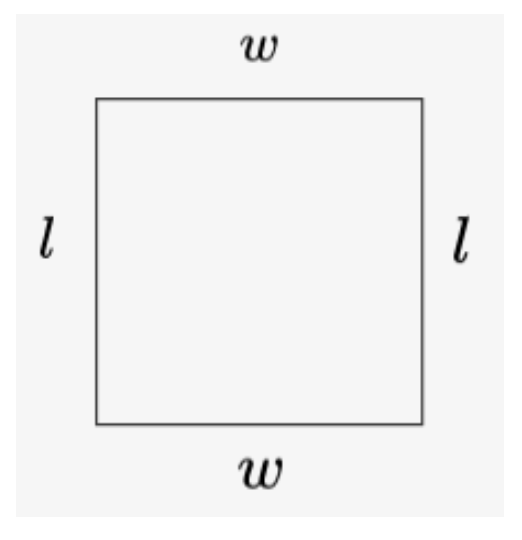To find the area, just multiply the length by the width. Which means our formula is…You’ll notice that we’re excluding some of the more “exotic” quadrilaterals here (e.g parallelogram, trapezoid, rhombus). There are formulas for the areas of these shapes, and you can feel free to look them up. However, you can always break any polygon you come across into rectangles and/or triangles, and then find the sum of the areas of each individual piece (see trapezoid below)!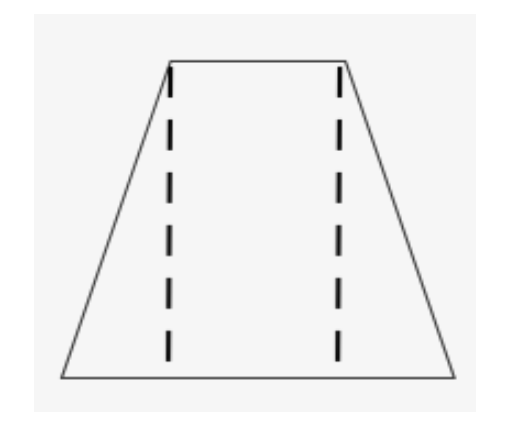## 2. Perimeter (of any polygon)

The perimeter describes the sum of all the sides in a polygon. So to find the perimeter of a triangle, add the three sides together. To find the perimeter of a rectangle, add the four sides together. Rather than memorize the individual perimeter formulas for each of these shapes, just use this “master” perimeter formula: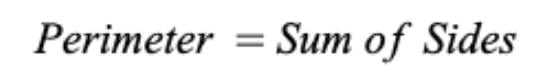That’s it.

## 3. Area of a triangle

Here’s one you might remember from high school. The formula for the area of a triangle is one-half the base times the height:Sounds easy enough, until you ask, “which side is the base? And what exactly is the height?”

The answer to the first question is very straightforward: Any side of the triangle can be used as a base. So in the picture below, why don’t we say that the base of triangle XYZ is side XZ.To find the height, go to the point that’s NOT on the base (in this case, Y), then draw a line down to create a right angle with the base (XZ). That’s your height. Let’s look at the same picture, but add in some numbers.If the base is 6 and the height is 4, then I’d calculate the area of the triangle by doing ½ * 6 * 4 = 12.

Obviously, this is a rather simple example. When you come across more difficult material, however, it’s extremely helpful to have a tutor who can walk you through problems step by step!

## 4. Pythagorean Theorem

An oldie but a goodie. This is a formula you learned back in 7th or 8th grade, and it’s probably still bouncing around in your noggin.

Have a look at the right triangle below, with sides A, B, and C.The two shorter sides, A and B, are known as the “legs” of the triangle, while the longest side, C, is the “hypotenuse.” These three sides in a right triangle will always be in the relationship…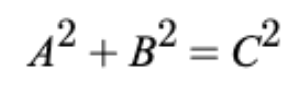Note: this does not apply to all triangles–just to right triangles

You have to admit, it is catchy. Old Pythagoras really knew how to write a zinger.

## 5. Area of a circle

To calculate the area of the circle, find the radius (distance from the center to the edge in the picture below), square it, and multiply it by π (approximately 3.14).This means that our formula is: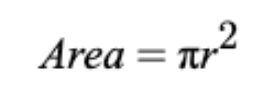It’s pretty easy to confuse this with the formula for circumference (below). To avoid doing that, think about this…what kind of units do you use in everyday life to measure area? You’d talk about the square footage of a house, or the square mileage of a town, right? So just remember that the formula with a square in it is area!

## 6. Circumference of a circle

The circumference of a circle is the distance all the way around the edge. To calculate it, multiply the radius by 2π.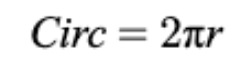## 7. Slope of a line

If you’ve ever been skiing, you know there’s a pretty significant difference between bunny slopes and black diamonds. Namely, the black diamonds are much steeper.

Well, to put an exact number on the “steepness” of a line on a graph, we use slope. Suppose we have two points, pictured on the graph below:To calculate the slope of the line between these two points, you’ll need the following formula: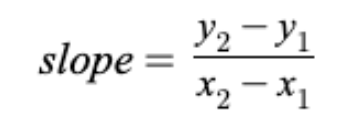However, if keeping all of those letters and numbers straight seems a little daunting, there’s an easier (and more alliterative) way to remember it:Simply find how high you “rise,” vertically from one point to another, and divide by how far you “run” horizontally. Let’s check this out with a specific example below.To calculate the slope of these two points, let’s first find the rise. The y-value of the first point is 2, and the y-value of the second point is 3, which means we “rose” 1. The x-value of the first point is 1 and the x-value of the second point is 3, so that’s a “run” of 2. Rise =1, Run = 2, so slope is ½.

## 8. Equation of a line:

Many of the GRE’s coordinate geometry questions deal with linear functions (that’s just a fancy way of saying “lines”).

Any line in the coordinate plane can be represented with the formula:In this formula, “m” represents the slope of the line, while b represents the “y-intercept.” Brings you right back to high school algebra class, doesn’t it? Let’s take a walk down memory lane with a specific example.

If we’re given the line y = 3x + 2, we immediately recognize that it matches the pattern above. In this case, the 3 is in the m-spot, so we can say that the slope of the line is 3 (or 3/1). Meanwhile, the b-value is 2, so our y-intercept is 2. What does that mean? Simply that the line hits the y-axis at 2. Thus, if we sketch this line, we’ll get the image below:## 9. Mean/average

To calculate the mean or average (both terms mean the same thing), you want to add up all the terms and divide by the number of terms. This means that the formula for finding the mean/average is:To illustrate with a specific example, if we want to find the average of the numbers 27, 32, 67, and 74, we would add them up (27 + 32 + 67 + 74 = 200), then divide that amount by the number of terms (4) to get 50.

All that said, many mean/average questions on the GRE—especially those in the quantitative comparison format—can often just be eyeballed.

For instance, suppose you were told that Class A had an average test score of 50, Class B had an average test score of 100, and the average of everyone in the two classes is 90. Which class has more students? Well, clearly Class B: If the two classes had an equal number of students, the overall average would have been right in the middle (75). But because it’s closer to Class B, we can assume that B has more people.

## 10. Distance, rate, and time

Colliding trains and high speed chases…Speed problems are by far the most action-packed questions the GRE has to offer. To succeed on them, though, you’ll need this basic formula: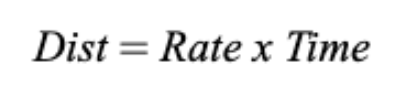To illustrate, if we’re travelling at a rate of 45mph for 2 hours, how far will we get? If we plug it into the formula, we’d get D =45 x 2, so 90 total miles is our distance.

## 11. Percent change

If you’re taking the GRE, you’re almost certainly an adult (unless this is some sort of Doogie Howser situation). As such, you probably work with percentages on a pretty regular basis. So if you have a system in your head that works for you, keep it. If not, here’s the formula to calculate the percent change between a new value and an old value.To use a specific example, if the price of a stock rises from \$40 to \$60, what is the percent increase? Well, in this case the “New Value” is 60 and the “Old Value” is 40. 60 minus 40 is 20, divided by 40 gives 0.5. Multiply that by 100 and I get 50. So the percent increase from 40 to 60 is 50%.

## 12. Volume of a 3-D figure

You might be asked to calculate the volume of a solid, such as the box in the figure below: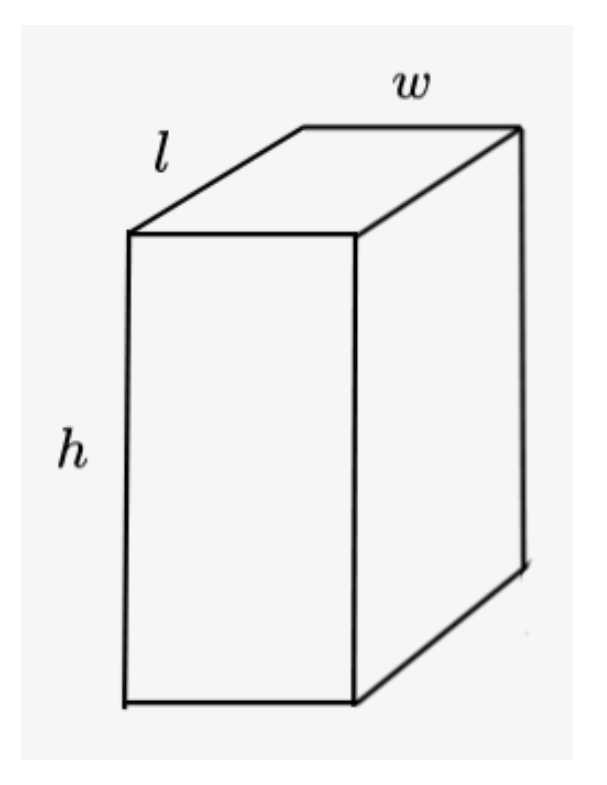If you are, the simplest formula to remember is…It’s true that you could remember the volume of a box as “length x width x height.” But the reason the above formula is nice is because it applies to other 3-D figures you might come across, like cylinders. In the case of a cylinder, the base is a circle. So you’d find the area of the circle, then multiply it by the height.

Note: You will not have to know how to calculate the volume of a pyramid, sphere, or cone. If those shapes pop up, you’ll be given the formula.

## A few top-shelf formulas (for 160+ test takers)

Of course, the above list is not exhaustive, but those are the formulas you’ll need if you’re looking to get a decent score. If you’re aiming even higher though, here are a few more:

### Permutations

If you have n items, and you want to count the number of ways to arrange r of them, use the following formula:### Combinations

If you have n items, and you want to count the number of ways to group r of them (that is, order does not matter), use the following formula:The generic format for a quadratic expression is:### Sum of interior angles

To calculate the sum of interior angles in a polygon with n sides, use the following formula: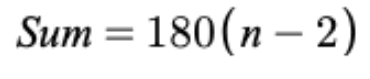For instance, a square has 4 sides, so the sum of interior angles is 180(4 – 2) = 360.

### Compound interest

To calculate the amount of money in a compound interest account that starts with P dollars, use the following formula (where r is the interest rate and t is the number of periods):## How to study for the GRE

It’s one thing to have these formulas memorized–it’s quite another to be able to apply them. In order to make them stick, it’s important to take GRE practice tests. By taking practice tests as part of your GRE math practice, you’ll not only be able to solidify the formulas, but you’ll also gain an understanding of the format and structure of the test. Your GRE quantitative score is determined by your performance on two quant sections. But here’s the kicker–the test adapts based on how you’re doing! If you do very well in the first section, the second one becomes harder. That’s not something you can simulate with a book of practice problems! Thus, it’s critical to include practice exams into your GRE prep.

Thanks for checking out this formula cheat sheet for the GRE Quantitative Reasoning section! As you continue to study, be sure to ask your GRE tutor any questions that arise!

### Latest Posts

#### What Beginners Need to Know About Stroke Order in Japanese Writing

A common term used in reference to Japanese writing is “stroke order”. Stroke order is...

#### The Importance of Tutoring: Enhancing Academic Success through Personalized Learning

Even in the earliest years of school students deal with constant pressures to excel academically,...

#### Understanding the Japanese-Language Proficiency Test (JLPT)

If you’ve been studying Japanese, you’ve likely heard of the “JLPT”. JLPT stands for “Japanese-Language...Making educational experiences better for everyone.

##### IXL

Comprehensive K-12 personalized learning

##### Rosetta Stone

Immersive learning for 25 languages

##### ABCya

Fun educational games for kids